Next: Hyperplanes and Duality Up: Projective Space Previous: Camera calibration:

## Projective Bases

A projective basis for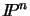is any set of n+2 points of, no n+1 of which lie in a hyperplane. Equivalently, the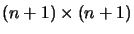matrix formed by the column vectors of any n+1 of the points must have full rank.

It is easily checked that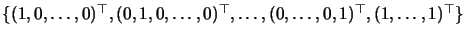forms a basis, called the canonical basis. It contains the points at infinity along each of the n coordinate axes, the origin, and the unit point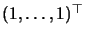. Any basis can be mapped into this standard form by a suitable collineation.

Property: A collineation onis defined entirely by its action on the points of a basis.

A full proof can be found in . We will just check that there are the right number of constraints to uniquely characterize the collineation. This is described by anmatrix A, defined up to an overall scale factor, so it has (n + 1)2-1= n(n+2) degrees of freedom. Each of the n+2 basis point images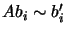provides n constraints (n+1 linear equations defined up a common scale factor), so the required total of n(n+2)constraints is met.

Exercise 2.3   : Consider three non-aligned points ai in the plane, and their barycenter g. Check that in homogeneous coordinates (x,y,1), we have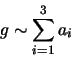An analogous relation holds for the unit point in the canonical basis.Next: Hyperplanes and Duality Up: Projective Space Previous: Camera calibration:
Bill Triggs
1998-11-13